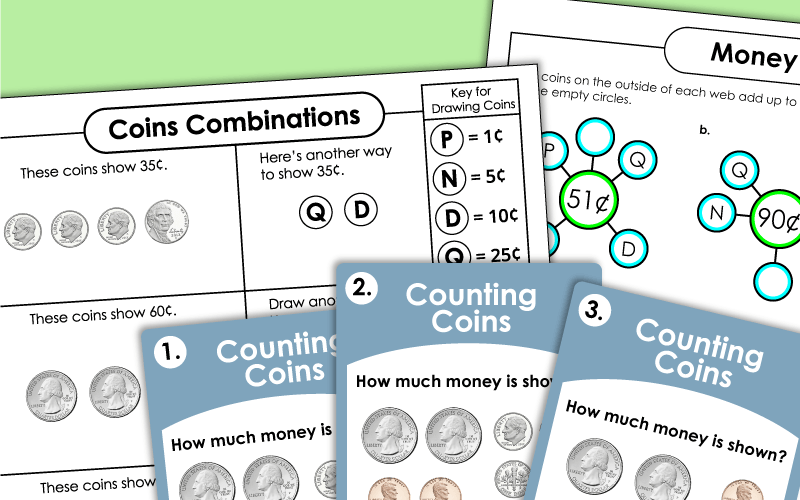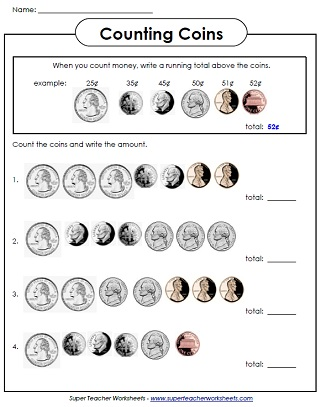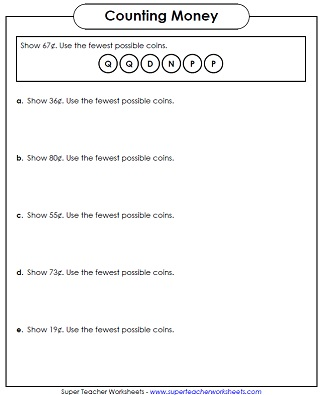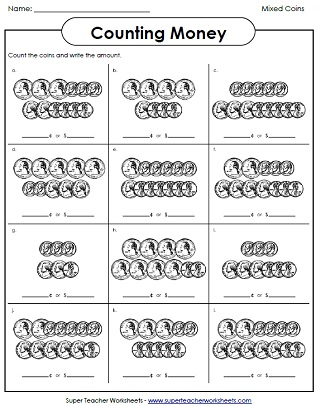Practice counting American coins with these printable worksheets, games, task cards, and activities. These advanced-level printables feature larger sets of mixed coins, and is intended for students in 3rd through 5th grades.There are many ways to use these printable puzzle pieces. Students will match each set of coins with the correct money amounts.
This file has 30 task cards with pictures of coins. Add up the values of the coins to find the totals.
Match the money amount to the coin cards in this memory game.  Hard level.
Players roll a die and collect coins.  The first player to collect a dollar's worth of coins is the winner.
Play this fun "coin counting" card game with your class.  Start a chain reaction as kids count the coins in the pictures and read the money amounts on their cards.

## WorksheetsCoins Only (No Bills)

Students use a running total to count a mixture of pennies, nickels, dimes, and quarters.
Count coins to buy items from the fruit stand.  Use the fewest number of coins possible.
Make the money amounts shown using the fewest number of coins possible.
Kids read the word problems and determine how much each person has.
Draw different coin combinations to show each money amount two ways.
Determine the amount of money shown. The use a different coin combination to show the same amount.
Convert the symbols to coins and add to find the total amount of money.
Use the chart to determine the value of letters and words.
A different version of the Money Words game that can be played alone or with a friend.
Count the groups of coins and write the amount.  Then, compare using the symbols: <, >, and =.
Many students have trouble writing money correctly.  Students must correctly write each money amount as dollars and cents and in word name form.

## Mixed Coins and Bills

Count the coins and bills (ones and fives) up to twelve dollars.
Money Totals  FREE
Students will figure out how much money each group of bills and coins is worth.

## Money Bond Worksheets

The coins on the outside of each web add up to the money total in the center circle. Write the correct coins in the empty circles.
This is a different variation of the money bonds worksheet above.
The coins on the outside of each web add up to one dollar. Write the correct coins in the empty circles.
Fill in the missing money amount for each number bond. Problems require subtraction.
Fill in the missing money amount for each number bond. Problems require subtraction.
Counting Mixed Coins (Basic)

If you're looking for more basic versions of the worksheets on this page, click here. These activities have fewer coins, and quarters are used sparingly.

More Money Worksheets

This page has worksheets on counting coins, operations with money, rounding money, and making change.

## Sample MoneyWorksheet PicturesMy Account
Site Information